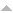Publications

Found 417 results
[ Author] Title Type Year
Filters: First Letter Of Last Name is A  [Clear All Filters]
A
Interior Symmetries and Multiple Eigenvalues for Homogeneous Networks. SIAM J. Appl. Dyn. Syst.. 2012;11.Edit
Denumerants of 3-numerical semigroups. In: Conference on Discrete Mathematics and Computer Science (Spanish). Vol 46. Elsevier Sci. B. V., Amsterdam; 2014. 3. p. 3-10p. (Electron. Notes Discrete Math.; vol 46).Edit
Factorization and catenary degree in 3-generated numerical semigroups. In: European Conference on Combinatorics, Graph Theory and Applications (EuroComb 2009). Vol 34. Elsevier Sci. B. V., Amsterdam; 2009. 1. p. 157-161p. (Electron. Notes Discrete Math.; vol 34).Edit
An algorithm to compute the primitive elements of an embedding dimension three numerical semigroup. In: Conference on Discrete Mathematics and Computer Science (Spanish). Vol 46. Elsevier Sci. B. V., Amsterdam; 2014. 1. p. 185-192p. (Electron. Notes Discrete Math.; vol 46).Edit
Factoring in embedding dimension three numerical semigroups. Electron. J. Combin.. 2010;17:Research Paper 138, 21.Edit
On the number of \$\ssfL\$-shapes in embedding dimension four numerical semigroups. Discrete Math.. 2015;338:2168-2178.Edit
Spectral refinement on quasi-diagonal matrices. Linear Algebra and its Applications. 2005;401:109-117.Edit
Spectral refinement for clustered eigenvalues of quasi-diagonal matrices. Linear Algebra and its Applications. 2006;413:394-402.Edit
Defect correction for spectral computations for a singular integral operator. Communications on Pure and Applied Analysis. 2006;5:241-250.Edit
An L1 refined projection approximate solution of the radiation transfer equation in stellar atmospheres. Journal of Computational and Applied Mathematics. 2002;140:13-26.Edit
Concentration inequalities for sequential dynamical systems of the unit interval. Ergodic Theory Dynam. Systems. 2016;36:2384-2407.Edit
Annealed and quenched limit theorems for random expanding dynamical systems. Probab. Theory Related Fields. 2015;162:233-274.Edit
Recurrence statistics for the space of interval exchange maps and the Teichmüller flow on the space of translation surfaces. Ann. Inst. Henri Poincaré Probab. Stat.. 2017;53:1371-1401.Edit
A note on the large deviations for piecewise expanding multidimensional maps. In: Nonlinear dynamics new directions. Vol 11. Springer, Cham; 2015. 1. p. 1-10p. (Nonlinear Syst. Complex.; vol 11).Edit
Polynomial loss of memory for maps of the interval with a neutral fixed point. Discrete Contin. Dyn. Syst.. 2015;35:793-806.Edit
Application of eigensolvers in quadratic eigenvalue problems for brake systems analysis. Vol 8584 LNCS Portugal, Guimaraes: Springer International Publishing 2014.
τ-complemented and τ-supplemented modules. Algebra Discrete Math.. 2006:1-16.Edit
The discrete Markus-Yamabe problem for symmetric planar polynomial maps. Indag. Math. (N.S.). 2012;23:603-608.